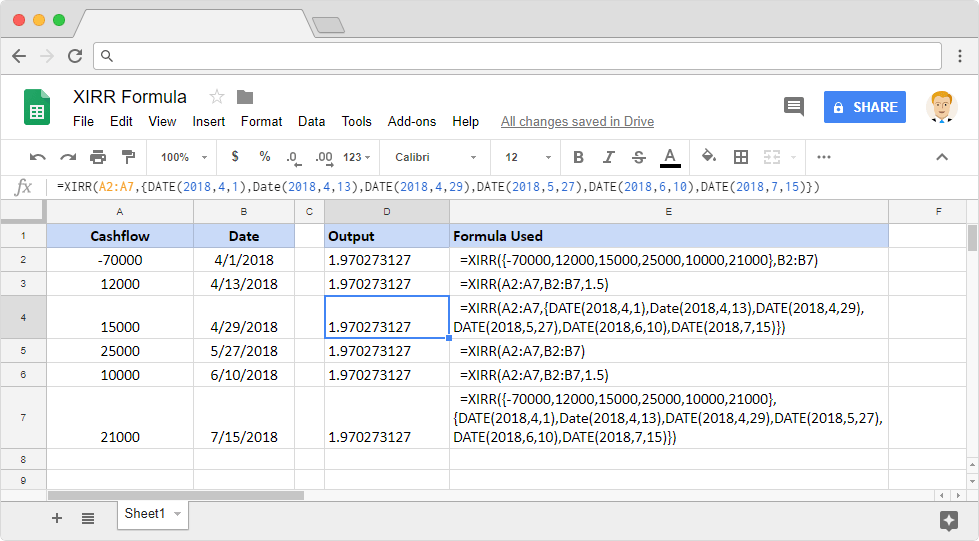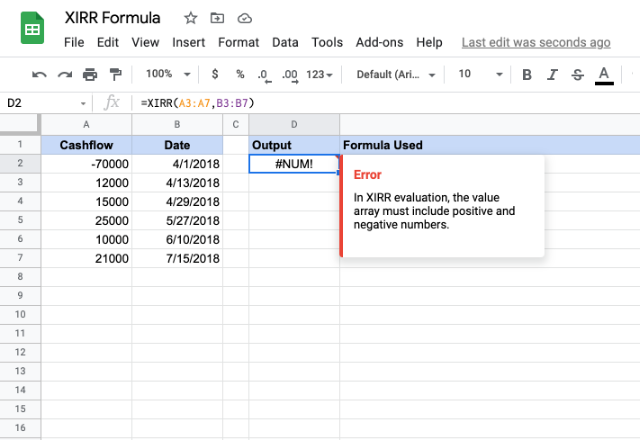# How to use the XIRR formula in Google SheetsDo you have a series of irregularly spaced cash flows of an investment? And you want to calculate the internal rate of return or annualized yield for that investment without the hassles of using complicated math? To our rescue, we have XIRR formula in Google Sheets. The internal rate of return (IRR) calculates the rate of return without involving external factors, such as inflation or the cost of capital. Hence the term ‘internal’. While IRR formula calculates the rate or the return for periodic cash flows, the XIRR formula does so for non-periodic cash flows.

### Syntax

XIRR(cashflow_amounts, cashflow_dates, [rate_guess])

• cashflow_amounts – is a group or range or array of incomes or payments corresponding to the investment. The input range of values for this parameter must contain at least one negative and one positive value. A negative value indicates that the buyer is investing, while a positive value indicates that the buyer is yielding a return.
• cashflow_dates – is the range of dates corresponding to the series of cash flows used in cashflow_amounts.
• rate_guess – [OPTIONAL – 0.1 by default] – is an estimated internal rate of return.

### Usage: XIRR formula in Google Sheets

Let us try and apply this formula practically so that we get a better hang of how things work with XIRR formula in Google Sheets. Please have a look at the snapshot below.As shown in multiple examples above, the cash flows can be direct numeric values or we can also use a reference to the range of cells that hold the cash flow values.

The input range of dates cannot be plain text values. Either they should be values returned by formulas such as DATE, TO_DATE etc. Or they can also be direct references to a range of cells that hold the dates.

What if we try the XIRR formula only with one directional cash flows? It coughs up a #NUM! error. That is because the XIRR formula needs both positive and negative cash flows for it to be able to calculate the rate of return.